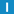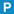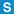# class Random

Library: Foundation
Package: Crypt

## Description

A better random number generator. Random implements a pseudo random number generator (PRNG). The PRNG is a nonlinear additive feedback random number generator using 256 bytes of state information and a period of up to 2^69.

## Member Summary

Member Functions: goodRand, initState, next, nextBool, nextChar, nextDouble, nextFloat, seed

## Enumerations

### Type

RND_STATE_0 = 8

linear congruential

RND_STATE_32 = 32

x**7 + x**3 + 1

RND_STATE_64 = 64

x**15 + x + 1

RND_STATE_128 = 128

x**31 + x**3 + 1

RND_STATE_256 = 256

x**63 + x + 1

## Constructors

### Random

Random(
int stateSize = 256
);

Creates and initializes the PRNG. Specify either a state buffer size (8 to 256 bytes) or one of the Type values.

## Destructor

### ~Random

~Random();

Destroys the PRNG.

## Member Functions

### nextUInt32 next();

Returns the next 31-bit pseudo random number.

### next

UInt32 next(
UInt32 n
);

Returns the next 31-bit pseudo random number modulo n.

### nextBoolbool nextBool();

Returns the next boolean pseudo random value.

### nextCharchar nextChar();

Returns the next pseudo random character.

### nextDoubledouble nextDouble();

Returns the next double pseudo random number between 0.0 and 1.0.

### nextFloatfloat nextFloat();

Returns the next float pseudo random number between 0.0 and 1.0.

### seed

void seed(
UInt32 seed
);

Seeds the pseudo random generator with the given seed.

### seed

void seed();

Seeds the pseudo random generator with a random seed obtained from a RandomInputStream.

### goodRandstatic UInt32 goodRand(
Int32 x
);

### initStatevoid initState(
UInt32 seed,
char * arg_state,
Int32 n
);